# ML 110: Poisoning by Gradients (30 pts extra)

## What You Need

• A Web browser

## Purpose

To practice using SecML, an open-source Python library for the security evaluation of Machine Learning algorithms.

In a browser, go to

From the menu, click File, "New notebook".

## Installing SecML

Execute these commands:
```!pip install secml import secml ```
The library installs, as shown below.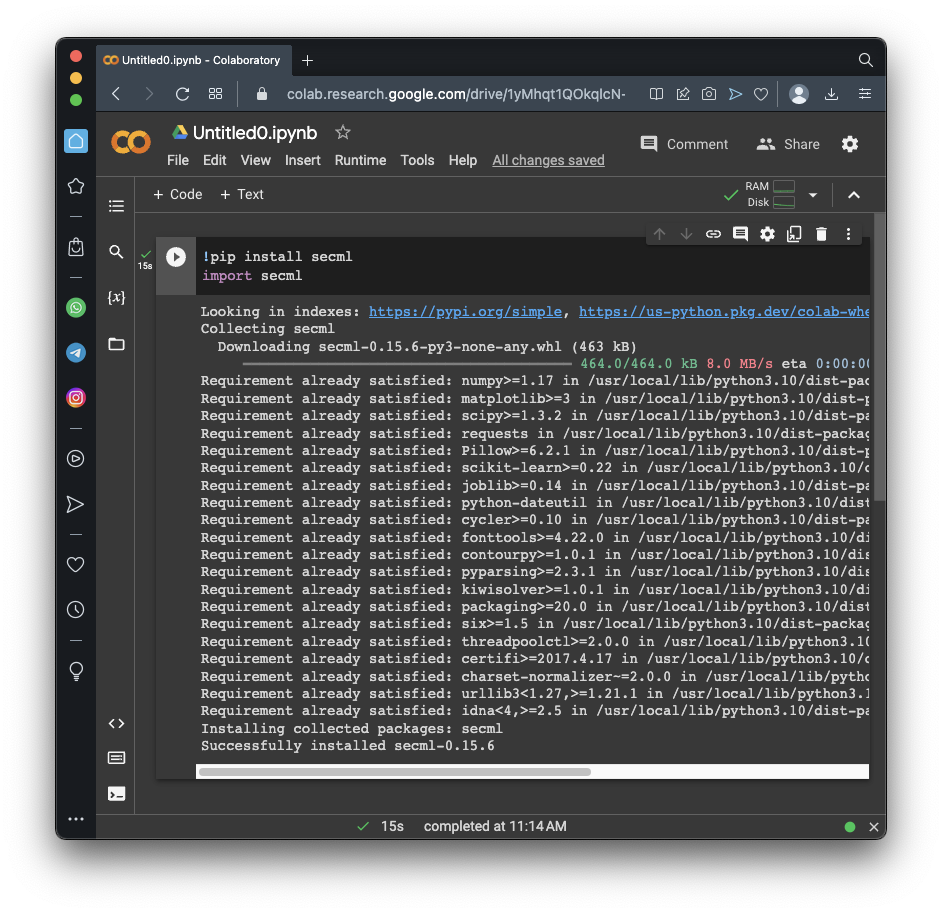## Preparing a Dataset

Execute these commands to create a simple, artificial dataset consisting of three groups of points in a plane.

We need training and testing sets, as usual. We also need a validation set, used to verify the classifier performance during the attack.

```random_state = 999 n_features = 2 # Number of features n_samples = 300 # Number of samples centers = [[-1, -1], [1, 1]] # Centers of the clusters cluster_std = 0.9 # Standard deviation of the clusters from secml.data.loader import CDLRandomBlobs dataset = CDLRandomBlobs(n_features=n_features, centers=centers, cluster_std=cluster_std, n_samples=n_samples, random_state=random_state).load() n_tr = 100 # Number of training set samples n_val = 100 # Number of validation set samples n_ts = 100 # Number of test set samples # Split in training, validation and test from secml.data.splitter import CTrainTestSplit splitter = CTrainTestSplit( train_size=n_tr + n_val, test_size=n_ts, random_state=random_state) tr_val, ts = splitter.split(dataset) splitter = CTrainTestSplit( train_size=n_tr, test_size=n_val, random_state=random_state) tr, val = splitter.split(dataset) # Normalize the data from secml.ml.features import CNormalizerMinMax nmz = CNormalizerMinMax() tr.X = nmz.fit_transform(tr.X) val.X = nmz.transform(val.X) ts.X = nmz.transform(ts.X) # Display the training set from secml.figure import CFigure # Only required for visualization in notebooks %matplotlib inline fig = CFigure(width=5, height=5) # Convenience function for plotting a dataset fig.sp.plot_ds(tr) fig.show() ```
As shown below, you see two categories of dots, shown in different colors.

The task of this model is to sort the dots into their categories.## Creating and Training the Model

Execute these commands to create and train the model:
```# Metric to use for training and performance evaluation from secml.ml.peval.metrics import CMetricAccuracy metric = CMetricAccuracy() # Creation of the multiclass classifier from secml.ml.classifiers import CClassifierSVM from secml.ml.kernels import CKernelRBF clf = CClassifierSVM(kernel=CKernelRBF(gamma=10), C=1) # We can now fit the classifier clf.fit(tr.X, tr.Y) print("Training of classifier complete!") # Compute predictions on a test set y_pred = clf.predict(ts.X) # Evaluate the accuracy of the classifier acc = metric.performance_score(y_true=ts.Y, y_pred=y_pred) print("Accuracy on test set: {:.2%}".format(acc)) ```
As shown below, the model has a 94% accuracy.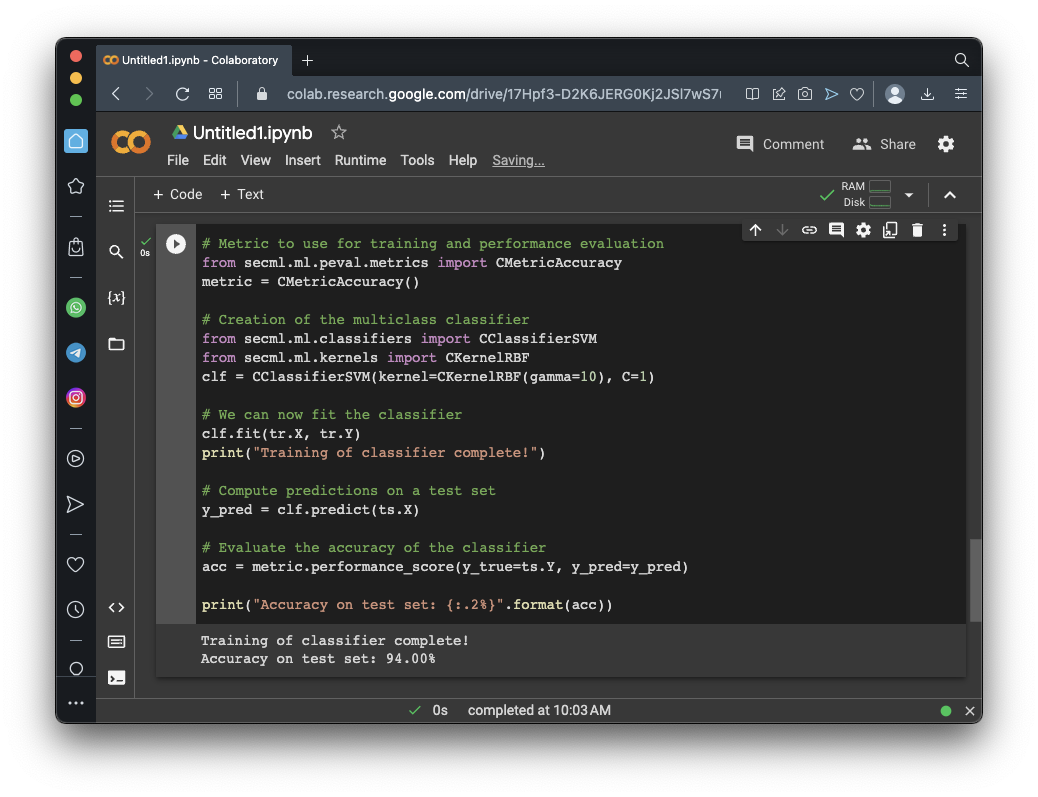## Visualizing the Results

Execute these commands to see a pretty cart showing the regions the model classifies data into:
```fig = CFigure(width=5, height=5) # Convenience function for plotting the decision function of a classifier fig.sp.plot_decision_regions(clf, n_grid_points=200) fig.sp.plot_ds(ts) fig.sp.grid(grid_on=False) fig.sp.title("Classification regions") fig.sp.text(0.01, 0.01, "Accuracy on test set: {:.2%}".format(acc), bbox=dict(facecolor='white')) fig.show() ```
As shown below, the model draws a curve between the regions, more or less the same way a human would.Execute these commands to preform a poisoning attack on a single point, and visualize it:
```lb, ub = val.X.min(), val.X.max() # Bounds of the attack space. Can be set to `None` for unbounded # Should be chosen depending on the optimization problem solver_params = { 'eta': 0.05, 'eta_min': 0.05, 'eta_max': None, 'max_iter': 100, 'eps': 1e-6 } from secml.adv.attacks import CAttackPoisoningSVM pois_attack = CAttackPoisoningSVM(classifier=clf, training_data=tr, val=val, lb=lb, ub=ub, solver_params=solver_params, random_seed=random_state) # chose and set the initial poisoning sample features and label xc = tr[0,:].X yc = tr[0,:].Y pois_attack.x0 = xc pois_attack.xc = xc pois_attack.yc = yc print("Before poisoning: ({:.2f}, {:.2f}), label: {}".format(xc.ravel().item(), xc.ravel().item(), yc.item())) from secml.figure import CFigure # Only required for visualization in notebooks %matplotlib inline fig = CFigure(4,5) grid_limits = [(lb - 0.1, ub + 0.1), (lb - 0.1, ub + 0.1)] fig.sp.plot_ds(tr) # highlight the initial poisoning sample showing it as a star fig.sp.plot_ds(tr[0,:], markers='*', markersize=16) fig.sp.title('Attacker objective and gradients') fig.sp.plot_fun( func=pois_attack.objective_function, grid_limits=grid_limits, plot_levels=False, n_grid_points=10, colorbar=True) # plot the box constraint from secml.optim.constraints import CConstraintBox box = fbox = CConstraintBox(lb=lb, ub=ub) fig.sp.plot_constraint(box, grid_limits=grid_limits, n_grid_points=10) n_poisoning_points = 1 # Number of poisoning points to generate pois_attack.n_points = n_poisoning_points pois_y_pred, pois_scores, pois_ds, f_opt = pois_attack.run(ts.X, ts.Y) fig.sp.plot_ds(pois_ds[0,:], markers='d', markersize=16) xp = pois_ds[0,:].X yp = pois_ds[0,:].Y print("After poisoning: ({:.2f}, {:.2f}), label: {}".format(xp.ravel().item(), xp.ravel().item(), yp.item())) fig.tight_layout() fig.show() ```
The chart shows the data points, with a background color showing the "Objective Function" -- a measure of the influence a point will have on the learning process.

The poisoning process moves the first point from the star, where it began, to the diamond shape above it, where the objective function is smaller.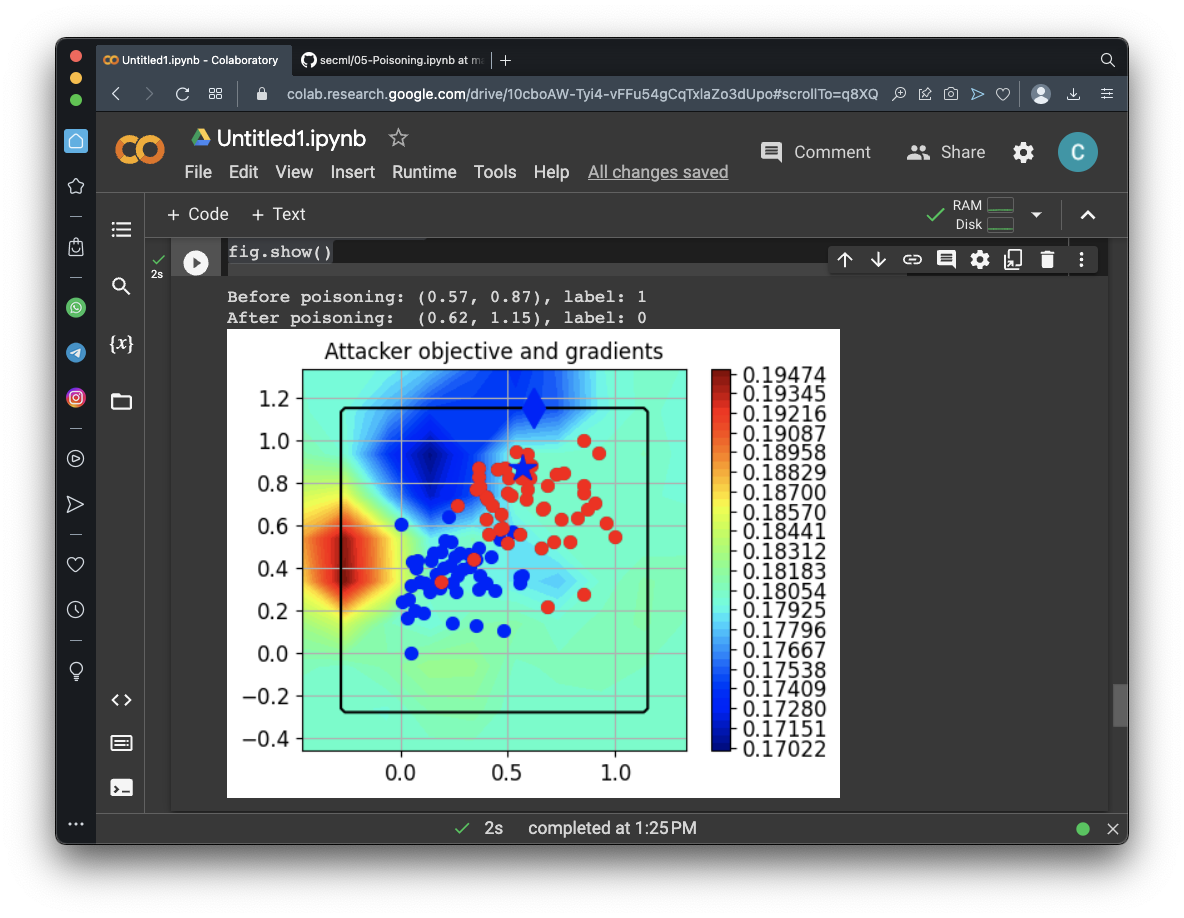## Performance of the Poisoned Model

Execute these commands to create and train the poisoned model, poisoning only one dot:
```n_poisoning_points = 1 # Number of poisoning points to generate pois_attack.n_points = n_poisoning_points # Run the poisoning attack print("Attack started...") pois_y_pred, pois_scores, pois_ds, f_opt = pois_attack.run(ts.X, ts.Y) print("Attack complete!") # Evaluate the accuracy of the original classifier acc = metric.performance_score(y_true=ts.Y, y_pred=y_pred) # Evaluate the accuracy after the poisoning attack pois_acc = metric.performance_score(y_true=ts.Y, y_pred=pois_y_pred) print("Original accuracy on test set: {:.2%}".format(acc)) print("Accuracy after attack on test set: {:.2%}".format(pois_acc)) ```
As shown below, the poisoned model has 93% accuracy.## Visualizing the Poisoning Process

Execute these commands to display the data before and after poisoning :
```# Training of the poisoned classifier pois_clf = clf.deepcopy() pois_tr = tr.append(pois_ds) # Join the training set with the poisoning points pois_clf.fit(pois_tr.X, pois_tr.Y) # Define common bounds for the subplots min_limit = min(pois_tr.X.min(), ts.X.min()) max_limit = max(pois_tr.X.max(), ts.X.max()) grid_limits = [[min_limit, max_limit], [min_limit, max_limit]] fig = CFigure(10, 10) fig.subplot(2, 2, 1) fig.sp.title("Original classifier (training set)") fig.sp.plot_decision_regions( clf, n_grid_points=200, grid_limits=grid_limits) fig.sp.plot_ds(tr, markersize=5) fig.sp.grid(grid_on=False) fig.subplot(2, 2, 2) fig.sp.title("Poisoned classifier (training set + poisoning points)") fig.sp.plot_decision_regions( pois_clf, n_grid_points=200, grid_limits=grid_limits) fig.sp.plot_ds(tr, markersize=5) fig.sp.plot_ds(pois_ds, markers='*', markersize=12) fig.sp.grid(grid_on=False) fig.subplot(2, 2, 3) fig.sp.title("Original classifier (test set)") fig.sp.plot_decision_regions( clf, n_grid_points=200, grid_limits=grid_limits) fig.sp.plot_ds(ts, markersize=5) fig.sp.text(0.05, -0.25, "Accuracy on test set: {:.2%}".format(acc), bbox=dict(facecolor='white')) fig.sp.grid(grid_on=False) fig.subplot(2, 2, 4) fig.sp.title("Poisoned classifier (test set)") fig.sp.plot_decision_regions( pois_clf, n_grid_points=200, grid_limits=grid_limits) fig.sp.plot_ds(ts, markersize=5) fig.sp.text(0.05, -0.25, "Accuracy on test set: {:.2%}".format(pois_acc), bbox=dict(facecolor='white')) fig.sp.grid(grid_on=False) fig.show() ```
As shown below, the top left chart shows the original data, and below it you see the trained model, with 94% accuracy.

On the right side, the top chart shows the data with a single poisining point (the star).

This point distorts the red-blue border at the top, near the added dot, but there's only one test data dot in that region, so it has only a minimal effect on the accuracy.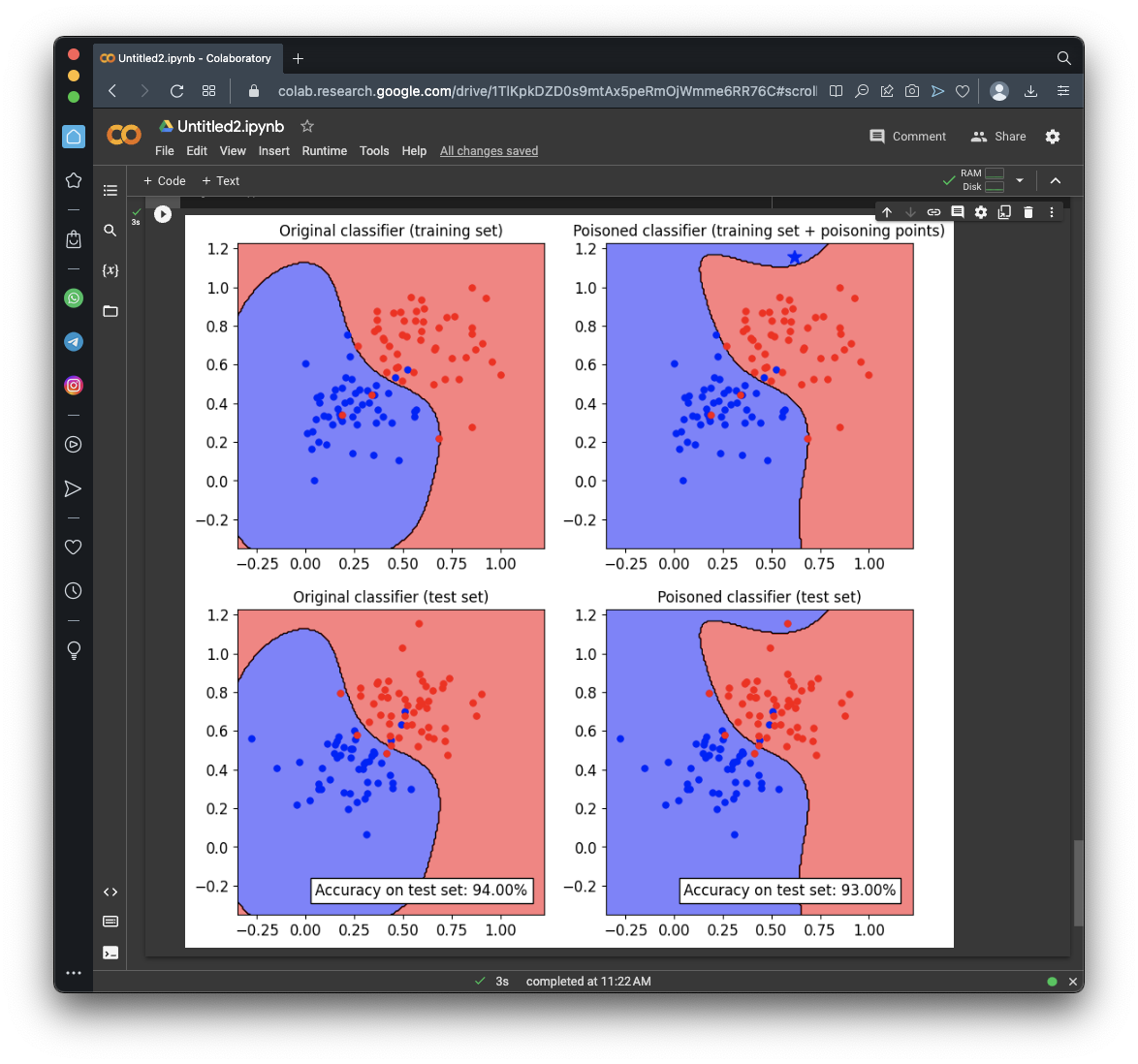## Flag ML 110.1: Poisoning More Points (10 pts)

Adjust the attack above to poison the first 20 dots in the training set.

The flag is covered by a green rectangle in the image below.## Flag ML 110.2: Poisoning all the Points (5 pts)

Adjust the attack above to poison all 100 dots in the training set.

The flag is covered by a green rectangle in the image below.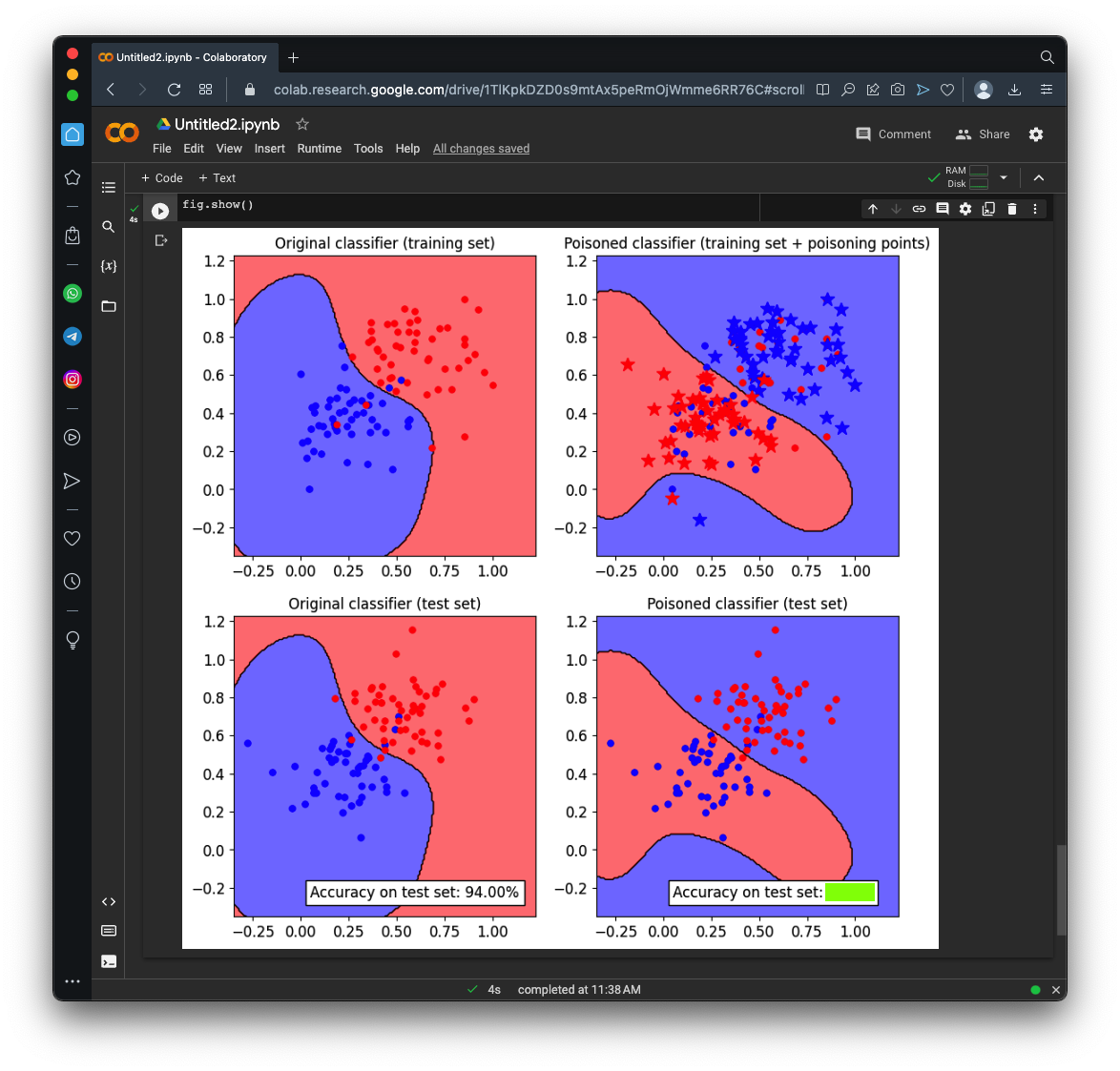## Flag ML 110.3: The Best Seed (15 pts)

Adjust the attack above to poison 5 dots.

There are three blue poison dots and two red ones, shown as stars in the image below.Examine the "Preparing a Dataset" code above. The seed for the pseudorandom number generator (random_state) was set to 999.

In the "Visualizing Gradient Descent" code, that same seed is used again in the pois_attack definition.

Change the seed in the "Visualizing Gradient Descent" code to 0. This will choose different poison points, as shown below.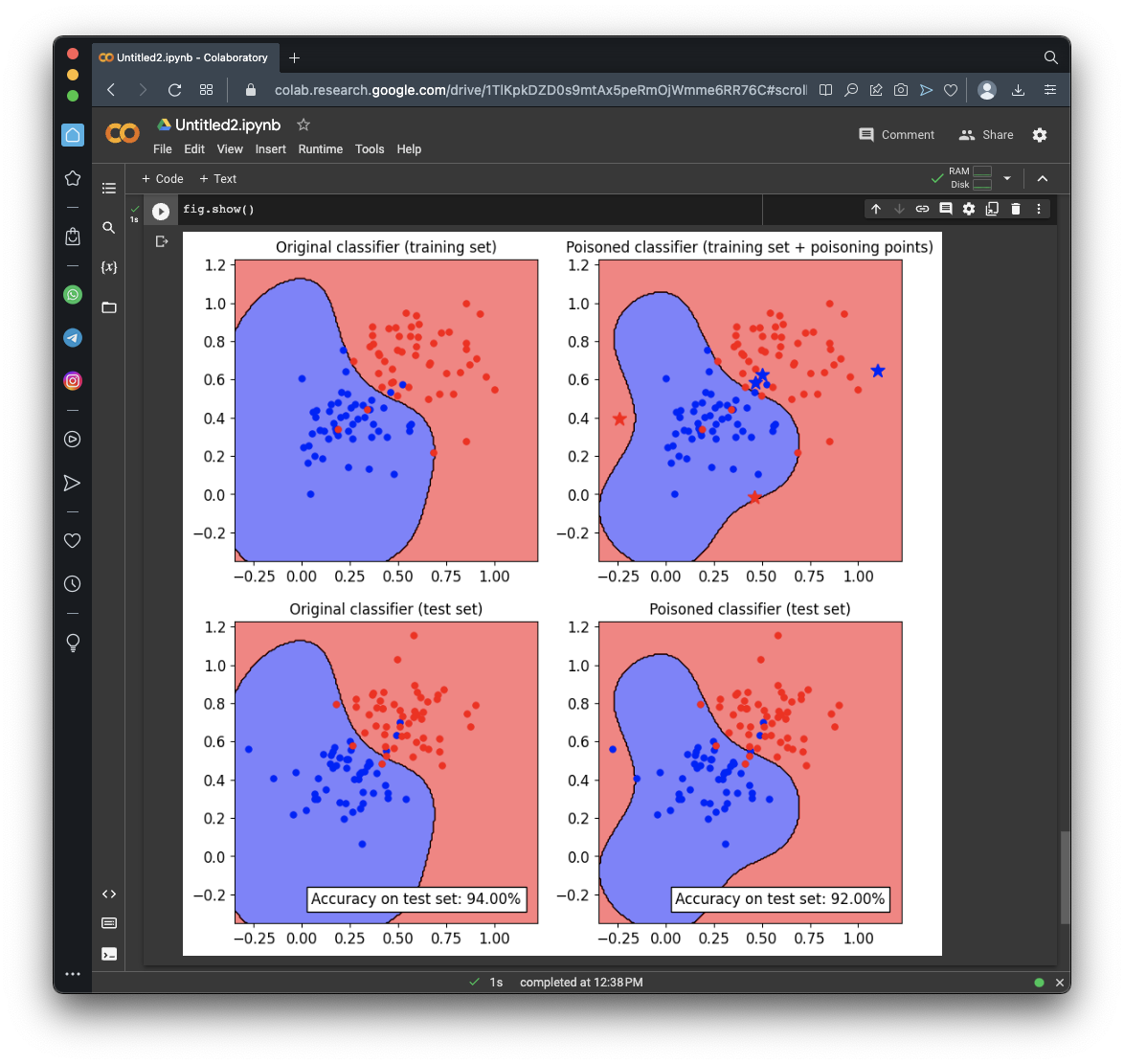Try seed values from 0 to 5. Use the seed that causes the largest decrease in performance.

The flag is covered by a green rectangle in the image below.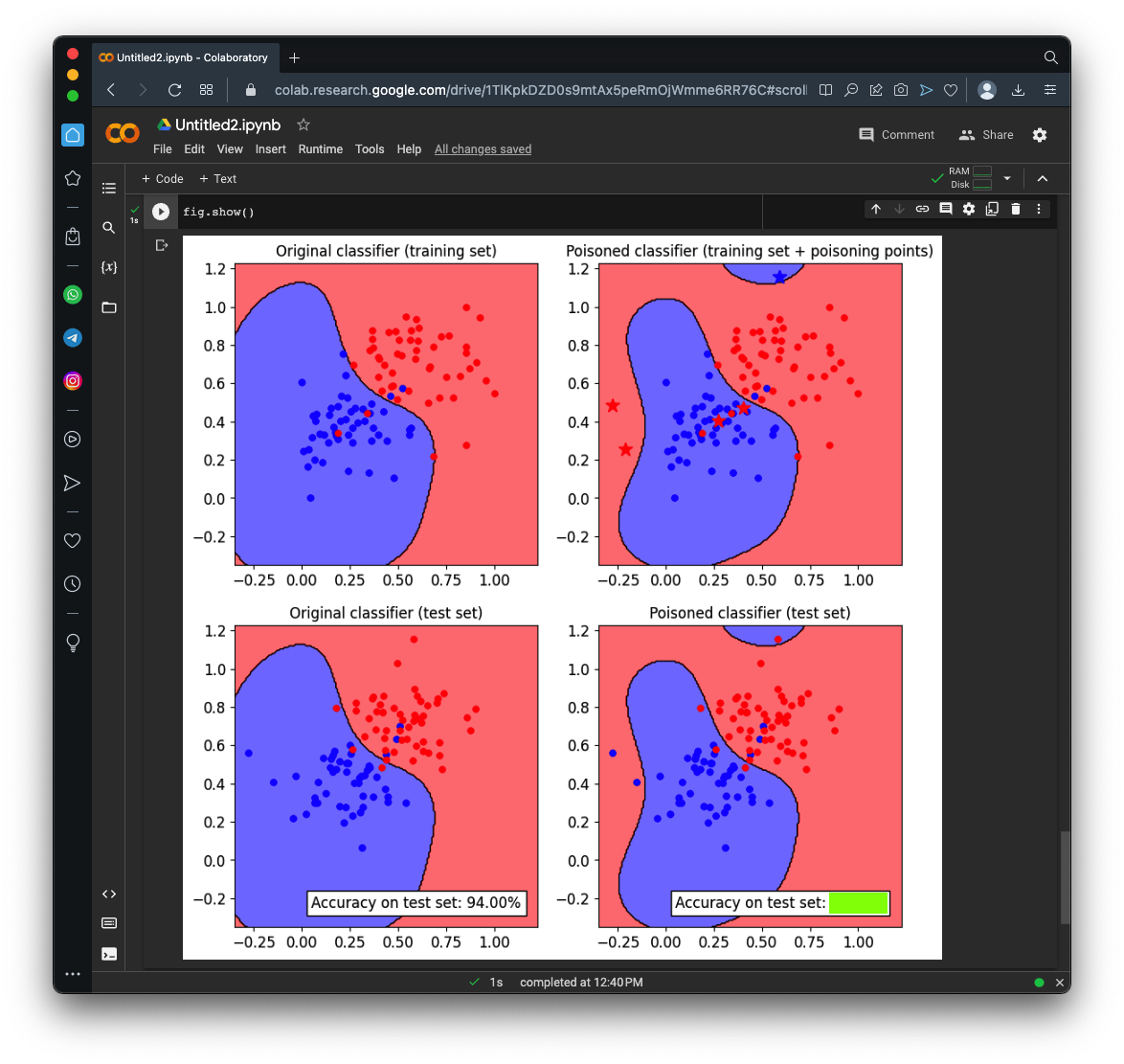## Sources

SecML: Secure and Explainable Machine Learning in Python
Poisoning Attacks against Machine Learning models
Poisoning attacks on Machine Learning

Posted 5-4-23